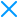October 13, 2014

# JavaScript and HTML5 Protection Questions 1

###### By Filipe Silva | 3 min readWe often find there are a number of questions that are common to many of our users. We will be collecting some of the more interesting and start sharing them here on our blog. Hopefully, they will help with your HTML5 and JavaScript code protection on Jscrambler too.

## How can I reach the maximum level of protection?

Our maximum level of protection is available both on our Professional and Ultimate plans. If you are doing something with HTML5, you’d be interested to know that we support protecting it, including Canvas code. These plans include also the Self-defending transformation, which is a combination of anti-tampering and anti-debugging. With the former, your code will be able to detect changes and break down intentionally, and the latter causes your code to break if debugging activities (e.g. popping up the Chrome Dev Console) are detected.

Regarding what protection to use, default templates such as “Obfuscation”, “Domain Lock” or “Self-defending” are solid, working out-of-the-box options to get your code protected. Further protecting your code works best if you have good knowledge of the original code. Are you trying to hide an algorithm? Prevent tampering? Hiding secrets? Depending on your answers, different transformations might be useful. With premium accounts, you can go to the Advanced Users tab and select the transformations individually that work best for your code.Figure 1 – Advanced Users Tab

Last but not least, you can also use the Ignore Code Blocks feature to increase substantially the protection in specific parts of your code, without growing your code too much or making the code visibly slower.

## Do you provide also non-alphanumeric obfuscation?

No, but we understand why you’re asking. Non-alphanumeric obfuscation is a visual nightmare and at first sight, it looks like something really hard to revert. However, the problem with full non-alphanumeric obfuscation is that the resulting code size is insane. As an example, something like ‘console.log(1)’ that has 15 bytes would end up as ~2500 bytes of non-alphanumeric code. If we apply this to an ordinary JavaScript, the resulting size would be unbearable. If that wasn’t enough, it is also rather easy to write a tool to automatically reverse this to its original form.

[][(![]+[])[+[]]+([![]]+[][[]])[+!+[]+[+[]]]+(![]+[])[!+[]+!+[]]+(!![]+[])[+[]]+(!![]+[])[!+[]+!+[]+!+[]]+(!![]+[])[+!+[]]][([][(![]+[])[+[]]+([![]]+[][[]])[+!+[]+[+[]]]+(![]+[])[!+[]+!+[]]+(!![]+[])[+[]]+(!![]+[])[!+[]+!+[]+!+[]]+(!![]+[])[+!+[]]]+[])[!+[]+!+[]+!+[]]+(!![]+[][(![]+[])[+[]]+([![]]+[][[]])[+!+[]+[+[]]]+(![]+[])[!+[]+!+[]]+(!![]+[])[+[]]+(!![]+[])[!+[]+!+[]+!+[]]+(!![]+[])[+!+[]]])[+!+[]+[+[]]]+([][[]]+[])[+!+[]]+(![]+[])[!+[]+!+[]+!+[]]+(!![]+[])[+[]]+(!![]+[])[+!+[]]+([][[]]+[])[+[]]+([][(![]+[])[+[]]+([![]]+[][[]])[+!+[]+[+[]]]+(![]+[])[!+[]+!+[]]+(!![]+[])[+[]]+(!![]+[])[!+[]+!+[]+!+[]]+(!![]+[])[+!+[]]]+[])[!+[]+!+[]+!+[]]+(!![]+[])[+[]]+(!![]+[][(![]+[])[+[]]+([![]]+[][[]])[+!+[]+[+[]]]+(![]+[])[!+[]+!+[]]+(!![]+[])[+[]]+(!![]+[])[!+[]+!+[]+!+[]]+(!![]+[])[+!+[]]])[+!+[]+[+[]]]+(!![]+[])[+!+[]]](([][(![]+[])[+[]]+([![]]+[][[]])[+!+[]+[+[]]]+(![]+[])[!+[]+!+[]]+(!![]+[])[+[]]+(!![]+[])[!+[]+!+[]+!+[]]+(!![]+[])[+!+[]]]+[])[!+[]+!+[]+!+[]]+(!![]+[][(![]+[])[+[]]+([![]]+[][[]])[+!+[]+[+[]]]+(![]+[])[!+[]+!+[]]+(!![]+[])[+[]]+(!![]+[])[!+[]+!+[]+!+[]]+(!![]+[])[+!+[]]])[+!+[]+[+[]]]+([][[]]+[])[+!+[]]+(![]+[])[!+[]+!+[]+!+[]]+(!![]+[][(![]+[])[+[]]+([![]]+[][[]])[+!+[]+[+[]]]+(![]+[])[!+[]+!+[]]+(!![]+[])[+[]]+(!![]+[])[!+[]+!+[]+!+[]]+(!![]+[])[+!+[]]])[+!+[]+[+[]]]+(![]+[])[!+[]+!+[]]+(!![]+[])[!+[]+!+[]+!+[]]+(+(+!+[]+[+!+[]]+(!![]+[])[!+[]+!+[]+!+[]]+[!+[]+!+[]]+[+[]])+[])[+!+[]]+(![]+[])[!+[]+!+[]]+(!![]+[][(![]+[])[+[]]+([![]]+[][[]])[+!+[]+[+[]]]+(![]+[])[!+[]+!+[]]+(!![]+[])[+[]]+(!![]+[])[!+[]+!+[]+!+[]]+(!![]+[])[+!+[]]])[+!+[]+[+[]]]+(+![]+[![]]+([]+[])[([][(![]+[])[+[]]+([![]]+[][[]])[+!+[]+[+[]]]+(![]+[])[!+[]+!+[]]+(!![]+[])[+[]]+(!![]+[])[!+[]+!+[]+!+[]]+(!![]+[])[+!+[]]]+[])[!+[]+!+[]+!+[]]+(!![]+[][(![]+[])[+[]]+([![]]+[][[]])[+!+[]+[+[]]]+(![]+[])[!+[]+!+[]]+(!![]+[])[+[]]+(!![]+[])[!+[]+!+[]+!+[]]+(!![]+[])[+!+[]]])[+!+[]+[+[]]]+([][[]]+[])[+!+[]]+(![]+[])[!+[]+!+[]+!+[]]+(!![]+[])[+[]]+(!![]+[])[+!+[]]+([][[]]+[])[+[]]+([][(![]+[])[+[]]+([![]]+[][[]])[+!+[]+[+[]]]+(![]+[])[!+[]+!+[]]+(!![]+[])[+[]]+(!![]+[])[!+[]+!+[]+!+[]]+(!![]+[])[+!+[]]]+[])[!+[]+!+[]+!+[]]+(!![]+[])[+[]]+(!![]+[][(![]+[])[+[]]+([![]]+[][[]])[+!+[]+[+[]]]+(![]+[])[!+[]+!+[]]+(!![]+[])[+[]]+(!![]+[])[!+[]+!+[]+!+[]]+(!![]+[])[+!+[]]])[+!+[]+[+[]]]+(!![]+[])[+!+[]]])[!+[]+!+[]+[+[]]]+(![]+[][(![]+[])[+[]]+([![]]+[][[]])[+!+[]+[+[]]]+(![]+[])[!+[]+!+[]]+(!![]+[])[+[]]+(!![]+[])[!+[]+!+[]+!+[]]+(!![]+[])[+!+[]]])[!+[]+!+[]+[+[]]]+[+!+[]]+(!![]+[][(![]+[])[+[]]+([![]]+[][[]])[+!+[]+[+[]]]+(![]+[])[!+[]+!+[]]+(!![]+[])[+[]]+(!![]+[])[!+[]+!+[]+!+[]]+(!![]+[])[+!+[]]])[!+[]+!+[]+[+[]]])()
Figure 2 – Non-alphanumeric example## Do you have something that cannot be reverse-engineered?

Everything can be reversed-engineered. So far the only thing that comes to our mind that has not been reversed engineered is the human brain. No solution can promise 100% protection.

That being said, our solution goes higher and deeper in providing you protection. It sets the difficulty level so high only a few percent of JS hackers will be able to reverse, and of those, even fewer will be motivated to do it.

Why? Because Jscrambler has more and better obfuscation techniques but contrary to other solutions, it goes beyond it. It leverages obfuscation to install code traps, scattered throughout the code that will provide you extra levels of protection:

• Licensing enforcing capabilities: Domain Lock, Browser/OS Lock, Expiration date
• Self-defending: Anti-tampering + Anti-debugging

That’s it for now! Keep visiting us because we’ll be covering other questions we come across and don’t forget to send us your questions, issues and suggestions to [email protected].

As a final word, don't forget to download our free data sheet on JavaScript Security Threats, which provides an overview of the most relevant attacks and how to prevent them.Filipe SilvaSoftware Engineer currently working as a Technical Product Manager leading Software Engineering and R&D teams.

## Subscribe to our weekly newsletter